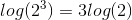# High School Math : Using Logarithms with Exponents

## Example Questions

### Example Question #2 : Simplifying Logarithms

Evaluate by hand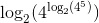Cannot be found by handExplanation:

Using the logarithm rules, exponents within logarithms can be removed and simply multiplied by the remaining logarithm.  This expression can be simplified as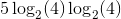### Example Question #7 : Simplifying Logarithms

Solve for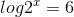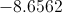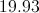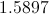Explanation:Use the power reducing theorem: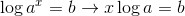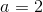and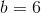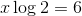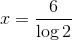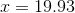### Example Question #1 : Simplifying Logarithms

Which of the following expressions is equivalent to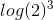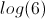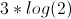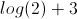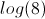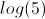Explanation:

According to the rule for exponents of logarithms,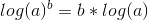. As a direct application of this,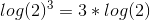.

### Example Question #9 : Simplifying Logarithms

Simplify the expression below.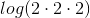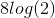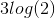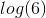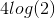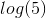Explanation:

Based on the definition of exponents,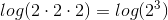.

Then, we use the following rule of logarithms: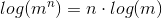Thus,